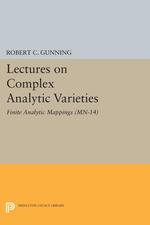# Lectures on Complex Analytic Varieties: Finite Analytic Mappings. (MN-14)

ROBERT C. GUNNING
Pages: 165
https://www.jstor.org/stable/j.ctt130hk90

1. Front Matter
(pp. None)
2. PREFACE
(pp. i-i)
R. C. Gunning
(pp. ii-ii)
4. §1. Finite analytic mappings
(pp. 1-37)

(a) These notes are intended as a sequel to the lecture notes [symbol], so it will be assumed from the outset that the reader is somewhat familiar with the contents of the earlier notes and the notation and terminology introduced in those notes will generally be used here without further reference. It will also be assumed that the reader has some background knowledge of the theory of functions of several complex variables and of the theory of sheaves, at least to the extent outlined at the beginning of the earlier notes. For clarity and emphasis however a brief introductory review...

5. §2. Finite analytic mappings with given domain
(pp. 38-85)

(a) Consider the problem of describing all finite analytic mappings from a given germ V of a complex analytic variety into another germ of complex analytic variety. The image of any such mapping is itself a germ of a complex analytic variety as a consequence of Theorem 2, so the mapping can be viewed as the composition of a surjective finite analytic mapping and an inclusion mapping; and the present interest centers on describing only the first of these two factors. If φ: V → W is a surjective finite analytic mapping then the induced homomorphism φ*:Wθ →Vθ...

6. §3. Finite analytic mappings with given range.
(pp. 86-143)

(a) Consider next the problem of describing all finite analytic mappings from germs of complex analytic varieties to a given germ V of a complex analytic variety. If φ: W → V is a finite analytic mapping the induced homomorphism φ*:Vθ →Wθ can be viewed as exhibitingWθ as a finitely generatedVθ-module; conversely ifWθ has the structure of a finitely generatedVθ-module then the mapping φ*:Vθ →Wθ defined by φ*(f) = f·1 ∈Wθ is clearly a finite homomorphism of complex algebras preserving the identities, and by Theorem 3(b) this is the homomorphism induced...

7. Appendix. Local cohomology groups of complements of complex analytic subvarieties.
(pp. 144-159)
8. INDEX OF SYMBOLS
(pp. 160-160)
9. INDEX
(pp. 161-163)
10. Back Matter
(pp. 164-164)